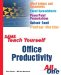# Why Use Formulas?

 Formulas are Excel's most powerful aid for getting your work done. Excel formulas handle the mathematical chores in your worksheet. In its simplest form, a formula is a quick calculation, similar to one you'd make on a calculator or adding machine. However, you can also use a formula to make predictions , figure out a car payment, or perform some other complex task. Formulas speed up the creation of your worksheets. You don't need to worry about whether a calculation is correct, because Excel doesn't make mistakes. Best of all, you can change any value contained in a formula, and Excel will update the results automatically.Sams Teach Yourself Office Productivity All in One (Sams Teach Yourself All in One)
ISBN: 0672325349
EAN: 2147483647
Year: 2003
Pages: 474
Authors: Greg Perry